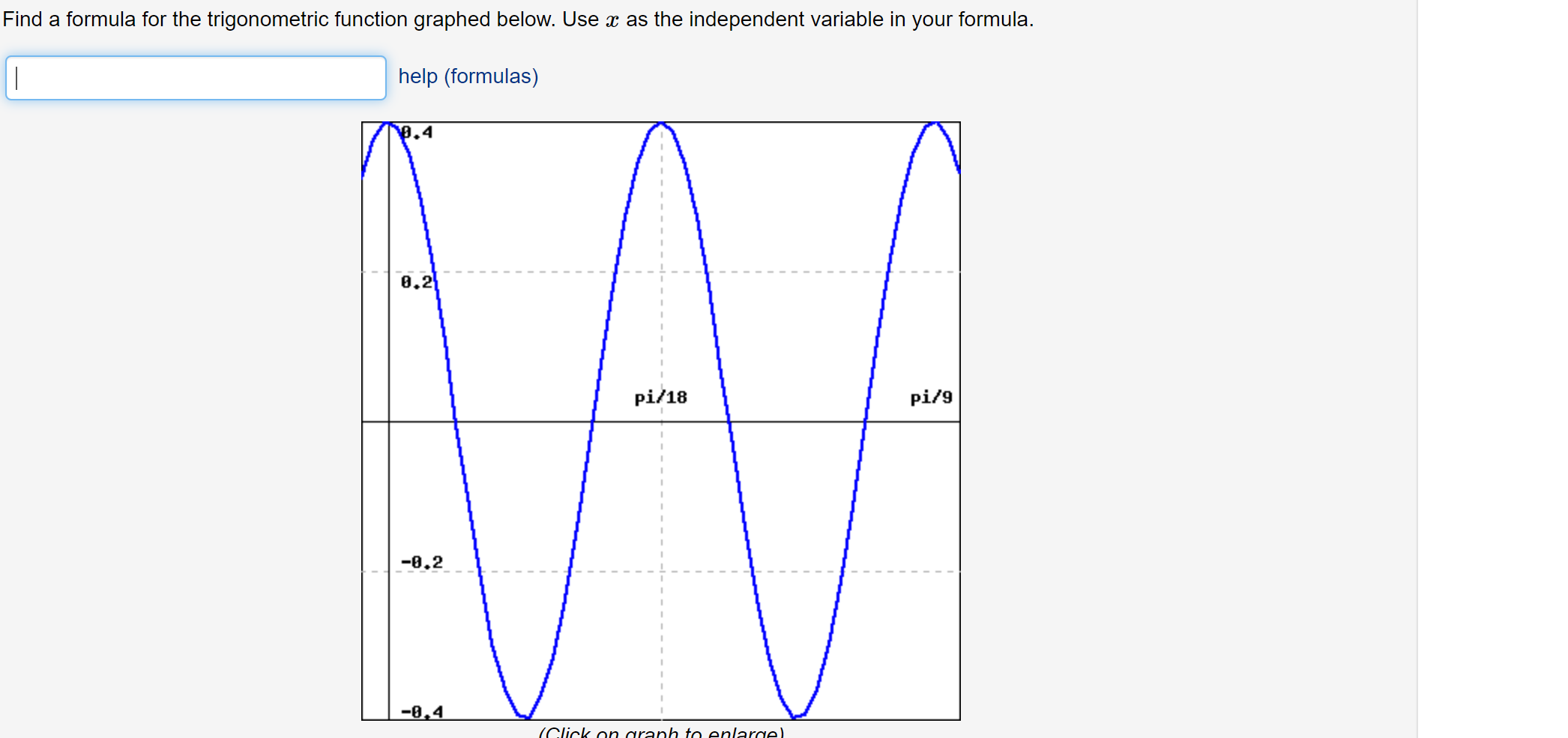# Find a formula for the trigonometric function graphed below. Use x as the independent variable in your formula.help (formulas).40.2pi/18pi/9-8.2-8,4(Click on araph to enlarge).

Question
31 viewshelp_outlineImage TranscriptioncloseFind a formula for the trigonometric function graphed below. Use x as the independent variable in your formula. help (formulas) .4 0.2 pi/18 pi/9 -8.2 -8,4 (Click on araph to enlarge). fullscreen
check_circle

Step 1

First find the parent function for the given curve, here the curve attains its maximum value at x = 0 and maximum value is 0.4, so parent function for this function is cosine function.

Let, the given function is of the form f(x) = A cos (B x) + C.

The Midline of the curve is y = 0, which is located in the middle of graphs maximum and minimum points, so the value of C = 0.

Step 2

The vertical distance between the midline and the maximum value attains at x = 0.4, So the value of amplitude A = 0.4.

...

### Want to see the full answer?

See Solution

#### Want to see this answer and more?

Solutions are written by subject experts who are available 24/7. Questions are typically answered within 1 hour.*

See Solution
*Response times may vary by subject and question.
Tagged in

### Other